# JEE Main 2020 Chemistry Paper With Solutions Sept 5 (Shift 1)

IIT JEE aspirants can refer to this page for the JEE Main 2020(Shift 1-5th Sep) Chemistry Question Paper with Solutions. These questions are solved by the experts at BYJU’S in the simplest possible method. Solving previous years’ question papers helps the students in understanding the coverage of the topics as well as the pattern of the paper.

### September 5 Shift 1 - Chemistry

1. The potential energy curve for the H2 molecule as a function of internuclear distance is: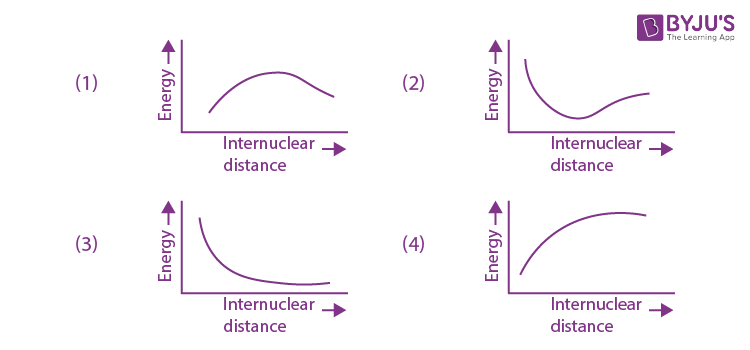Solution:

1. Solution: (2)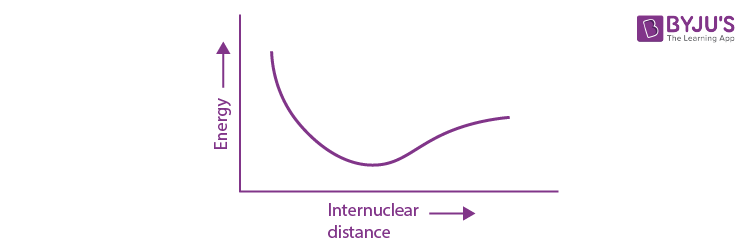2. The most appropriate reagent for conversion of C2H5CN into CH3CH2CH2NH2 is:

1. 1) NaBH4
2. 2) Na(CN)BH3
3. 3) CaH2
4. 4) LiAlH4

Solution:

1.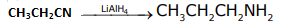Solution:(4)

3. Which of the following is not an essential amino acid?

1. 1) Valine
2. 2) Tyrosine
3. 3) Lysine
4. 4) Leucine

Solution:

1. Tyrosine in not an essential amino acid

Solution : (2)

4. Which of the following derivatives of alcohols is unstable in an aqueous base?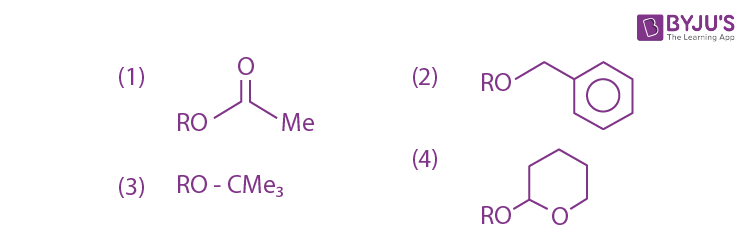Solution:

1. Hydrolysis of ester occurs in basic medium.

Solution: (1)

5. The structure of PCl5 in the solid state is:

1. 1) Square planar [PCl4]+ and octahedral [PCl6]
2. 2) Tetrahedral [PCl4]+ and octahedral [PCl6]
3. 3) Trigonal bipyramidal
4. 4) Square pyramidal

Solution:

1. In solid state PCl5 exist in Ionpair i.e. (PCl4+) and (PCl6)

PCl4+ (sp3tetrahedral)

PCl6(sp3d2) – octahedral)

Solution: (2)

6. A diatomic molecule X2 has a body-centred cubic (bcc) structure with a cell edge of 300 pm. The density of the molecule is 6.17 g cm–3. The number of molecules present in 200 g of X2 is:(Avogadro constant (NA) = 6 × 1023 mol-1)

1. 1) 8 NA
2. 2) 2 NA
3. 3) 40 NA
4. 4) 4 NA

Solution:

1. X2 = BCC

a = 300pm

d= 6.17 g/cm3=

$$\frac{2\times GMM}{6\times 10^{23}\times (300\times 10^{-10})^{3}}$$

$$GMM = \frac{6.17\times 6\times 9\times 3\times 10^{-1}}{2}$$

GMM = 81 x 6.17 x 10-1 =49.97 g/mol

Number of molecules = 200g/49.97g/mol= 4mol

=4NA

Solution: (4)

7. The equation that represents the water-gas shift reaction is: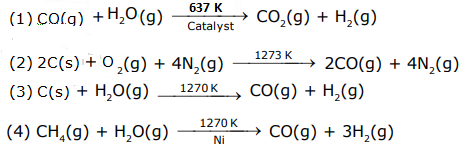Solution:

1. Solution: (1)

Fact

8. The increasing order of the acidity of the α-hydrogen of the following compounds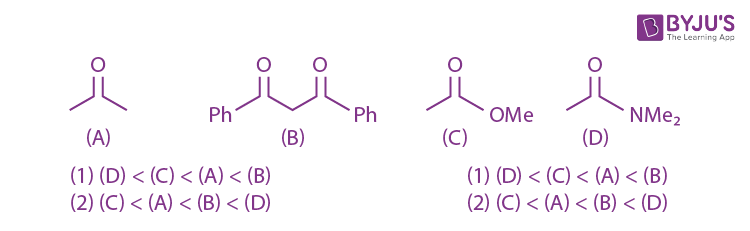Solution:

1. Stability order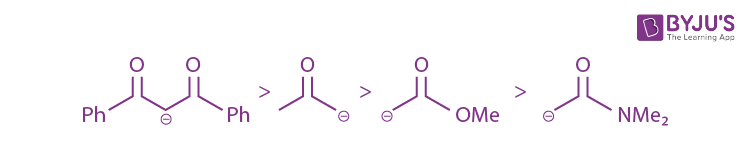Solution: (1)

9.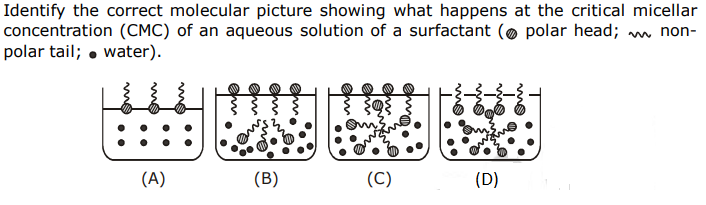1. 1) (B)
2. 2) (A)
3. 3) (C)
4. 4) (D)

Solution:

1.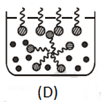Solution: (4)

10. If a person is suffering from the deficiency of nor-adrenaline, what kind of drug can be suggested?

1. 1) Antihistamine
2. 2) Antidepressant
3. 3) Anti-inflammatory
4. 4) Analgesic

Solution:

1. If nor-adrenaline is low, person may suffer from depression. Hence, anti depressant drug is suggested.

Solution: (2)

11. The values of the crystal field stabilization energies for a high spin d6 metal ion in octahedral and tetrahedral fields, respectively, are:

1. 1) –2.4 Δo and –0.6 Δt
2. 2) –1.6 Δo and –0.4 Δt
3. 3) –0.4 Δo and –0.27 Δt
4. 4) –0.4 Δo and –0.6 Δt

Solution:

1. d6(octahedral) = high spin complex

= t2g4 eg2

$$CFSE = \left [ -\frac{2}{5}\times 4+\frac{3}{5}\times 2 \right ]\Delta _{0}$$

$$CFSE = \left [ \frac{-8+6}{5} \right ]\Delta _{0}$$

$$= -0.4\Delta _{0}$$

d6 (tetrahedral) = high spin complex

= t2g3 eg3

$$CFSE = \left [ -\frac{3}{5}\times 3+\frac{2}{5}\times 3 \right ]\Delta _{t}$$

$$= -0.6\Delta _{t}$$

Solution: (4)

12. A flask contains a mixture of compounds A and B. Both compounds decompose by first order kinetics. The half-lives for A and B are 300 s and 180 s, respectively. If the concentrations of A and B are equal initially, the time required for the concentration of A to be four times that of B (in s) is: (Use ln 2 = 0.693)

1. 1) 180
2. 2) 300
3. 3) 120
4. 4) 900

Solution:

1. At = A0 .e-k1t

Bt = B0.e-k2t

k1=ln2/300

k2 =ln2/180

At and Bt are related as [A] = 4[B] A0 .e-k1t= 4 x B0.e-k2t

(t/180) – (t/300) =2

(t/3) – (t/5) =120

(2t/15)=120

t=900 sec

Solution: (4)

13. The increasing order of basicity of the following compounds is: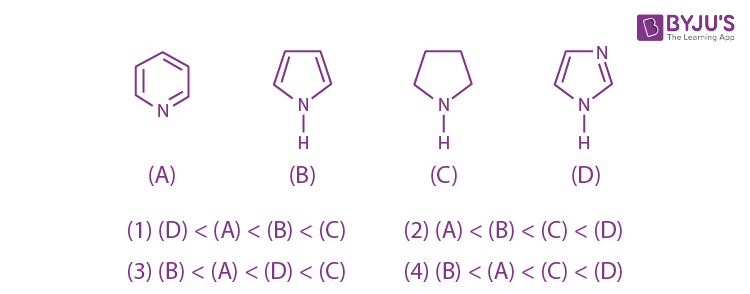Solution:

1. Correct order of basicity.Solution: (4)

14. The condition that indicates a polluted environment is:

1. 1) pH of rain water to be 5.6
2. 2) BOD value of 5 ppm
3. 3) 0.03% of CO2 in the atmosphere
4. 4) eutrophication

Solution:

1. Eutrophication is the condition in which excessive richness of nutrients in a lake or water body, which causes dense growth of plant life and BOD increases.

Solution: (4)

15. In the sixth period, the orbitals that are filled are:

1. 1) 6s, 5d, 5f, 6p
2. 2) 6s, 4f, 5d, 6p
3. 3) 6s, 6p, 6d, 6f
4. 4) 6s, 5f, 6d, 6p

Solution:

1. energy order of orbital’s according to Aufbau principle

6s < 4f < 5d < 6p

Solution: (2)

16. The difference between the radii of 3rd and 4th orbits of Li2+ is ΔR1. The difference between the radii of 3rd and 4th orbits of He+ is ΔR2. Ratio ΔR1: ΔR2 is:

1. 1) 8 : 3
2. 2) 3 : 8
3. 3) 3 : 2
4. 4) 2 : 3

Solution:

1. $$(R_{4}-R_{3})_{Li^{+2}}=\frac{0.529}{3}\left \{ 4^{2}-3^{2} \right \}=\Delta R_{1}$$

$$(R_{4}-R_{3})_{He^{+2}}=\frac{0.529}{2}\left \{ 4^{2}-3^{2} \right \}=\Delta R_{2}$$

$$\frac{\Delta R_{1}}{\Delta R_{2}}=\frac{1/3}{1/2}=\frac{2}{3}$$

Solution: (4)

17. In the following reaction sequence the major products A and B are: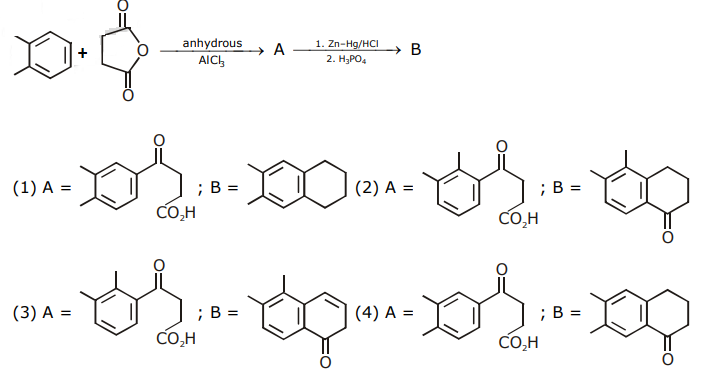Solution:

1.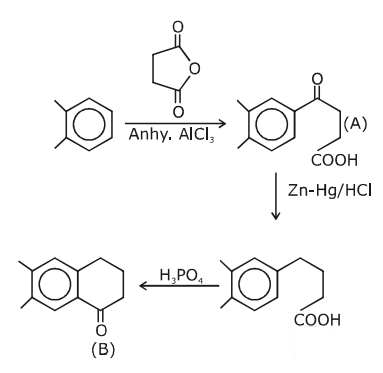Solution: (4)

18: The correct electronic configuration and spin-only magnetic moment (BM) of Gd3+ (Z = 64), respectively, are:

1. 1) [Xe] 5f7 and 7.9
2. 2) [Xe] 4f7 and 7.9
3. 3) [Xe] 5f7 and 8.9
4. 4) [Xe] 4f7 and 8.9

Solution:

1.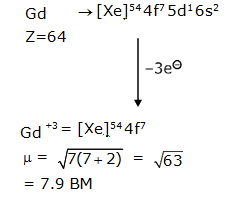Solution: (2)

19: An Ellingham diagram provides information about:

1. 1) The pressure dependence of the standard electrode potentials of reduction reactions involved in the extraction of metals.
2. 2) The conditions of pH and potential under which a species is thermodynamically stable.
3. 3) The kinetics of the reduction process.
4. 4) The temperature dependence of the standard Gibbs energies of formation of some metal oxides.

Solution:

1. Solution: (4)

Fact

20. Consider the following reaction: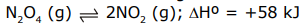For each of the following cases (a, b), the direction in which the equilibrium shifts is:

(a) Temperature is decreased.

(b) Pressure is increased by adding N2 at constant T.

1. 1) (a) towards reactant, (b) towards product
2. 2) (a) towards reactant, (b) no change
3. 3) (a) towards product, (b) towards reactant
4. 4) (a) towards product, (b) no change

Solution:

1.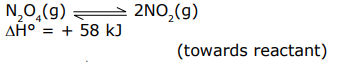(a) Temperature decreases implies Backward shift as it is endothermic reaction

(b) As ‘N2’ will not react with both N2O4 & NO2, as moles increases in reactants, as much as in products, a = hence there is no change in equilibrium.

Therefore no change

Solution: (2)

21: The minimum number of moles of O2 required for complete combustion of 1 mole of propane and 2 moles of butane is _____.

Solution:

1.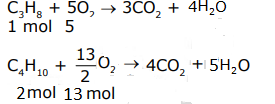Total required mol of O2 = 5 + 13 = 18

22. The number of chiral carbon(s) present in piptide, Iie-Arg-Pro, is ______ .

Solution:

1.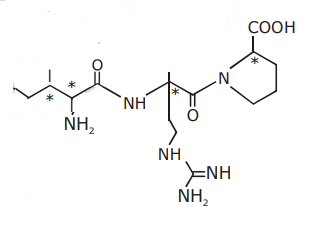Solution:(4)

23. A soft drink was bottled with a partial pressure of CO2 of 3 bar over the liquid at room temperature. The partial pressure of CO2 over the solution approaches a value of 30 bar when 44 g of CO2 is dissolved in 1 kg of water at room temperature. The approximate pH of the soft drink is ______ × 10–1

(First dissociation constant of H2CO3= 4.0 × 10–7; log 2 = 0.3; density of the soft drink= 1 g mL–1)

Solution:

1. $$CO_{2}+H_{2}O\rightarrow H_{2}CO_{3}$$

30 bar = 1 m/lit

3 bar = 0.1 m/lit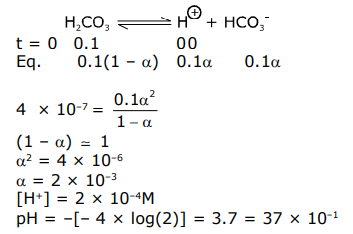Solution: (37)

24. An oxidation-reduction reaction in which 3 electrons are transferred has a ΔGº of 17.37 kJmol–1 at 250C. The value of E0cell (in V) is ______ × 10–2. (1 F = 96,500 C mol–1)

Solution:

1. ΔG° = –nFE°

17.37 × 1000 = –3 × 96500 × E°

E° = 17370/(3x96500)

E° = 579/9650 volt

= 0.06 = 6 × 10–2 volt

Solution: (6)

25. The total number of coordination sites in ethylenediaminetetraacetate (EDTA4–) is _____.

Solution:

Solution: (6)

### JEE Main 2020 Chemistry Paper With Solutions Shift 1 September 5